One-Hot Encoding a Feature on a Pandas Dataframe: Examples

# One-Hot Encoding a Feature on a Pandas Dataframe: Examples

Last updated:

Updated for Pandas 1.0

Dummy encoding is not exactly the same as one-hot encoding. For more information, see Dummy Variable Trap in regression models

When extracting features, from a dataset, it is often useful to transform categorical features into vectors so that you can do vector operations (such as calculating the cosine distance) on them.

All examples available on this notebook

Think about it for a second: how would you naïvely calculate the distance between users using the cosine difference, where their country of origin is the only feature?

You need a way that will correctly return zero for users that share the same country and 1 (maximum) for users that don't.

How would you calculate the distance between users in a dataset, where their country of origin is the only feature?

Take this dataset for example:

+---+----------------+
| id| country        |
+---+----------------+
|  0| russia         |
|  1| germany        |
|  2| australia      |
|  3| korea          |
|  4| germany        |
+---+----------------+


One of the ways to do it is to encode the categorical variable as a one-hot vector, i.e. a vector where only one element is non-zero, or hot.

With one-hot encoding, a categorical feature becomes an array whose size is the number of possible choices for that features, i.e.:

+---+---------------+----------------+------------------+---------------+
| id| country=russia| country=germany| country=australia|  country=korea|
+---+---------------+----------------+------------------+---------------+
|  0|              1|               0|                 0|              0|
|  1|              0|               1|                 0|              0|
|  2|              0|               0|                 1|              0|
|  3|              0|               0|                 0|              1|
|  4|              0|               1|                 0|              0|
+---+---------------+----------------+------------------+---------------+


## One-hot encode column

To create a dataset similar to the one used above in Pandas, we could do this:

import pandas as pd

df = pd.DataFrame({'country': ['russia', 'germany', 'australia','korea','germany']})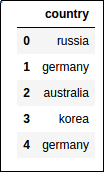original-dataframe

Pandas provides the very useful get_dummies method on DataFrame, which does what we want:

import pandas pd

df = pd.DataFrame({'country': ['russia', 'germany', 'australia','korea','germany']})
pd.get_dummies(df['country'], prefix='country')original dataframe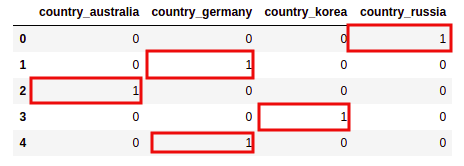one-hot-encoded-features

## One-hot encoding vs Dummy variables

By default, the get_dummies() does not do dummy encoding, but one-hot encoding.

To produce an actual dummy encoding from your data, use drop_first=True (not that 'australia' is missing from the columns)

import pandas as pd

# using the same example as above
df = pd.DataFrame({'country': ['russia', 'germany', 'australia','korea','germany']})

pd.get_dummies(df["country"],prefix='country',drop_first=True)original dataframe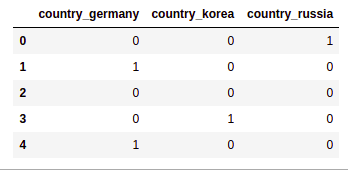No australia for you!
Some machine learning techniques require you
to drop one dimension from the representation so as
to avoid dependency among the variables.
Use "drop_first=True" to achieve that.

## Columns for categories that only appear in test set

You need to inform pandas if you want it to create dummy columns for categories even though never appear (for example, if you one-hot encode a categorical variable that may have unseen values in the test).

Use .astype(<col-name>, CategoricalDtype([<list-of-categories>])):

import pandas as pd

from pandas.api.types import CategoricalDtype

# say you want a column for "japan" too (it'll be always zero, of course)
df["country"] = train_df["country"].astype(CategoricalDtype(["australia","germany","korea","russia","japan"]))

# now call .get_dummies() as usual
pd.get_dummies(df["country"],prefix='country')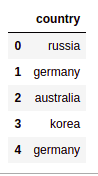BEFORE: source dataframe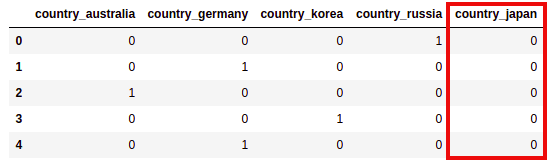AFTER: a column was created for japan
(all zeros) even though
it did not appear as
a value in the source dataframe

## Add dummy columns to dataframe

All in one line: df = pd.concat([df,pd.get_dummies(df['mycol'], prefix='mycol',dummy_na=True)],axis=1).drop(['mycol'],axis=1)

For example, if you have other columns (in addition to the column you want to one-hot encode) this is how you replace the country column with all 3 derived columns, and keep the other one:

Use pd.concat() to join the columns and then drop() the original country column:

import pandas as pd

# df now has two columns: name and country
df = pd.DataFrame({
'name': ['josef','michael','john','bawool','klaus'],
'country': ['russia', 'germany', 'australia','korea','germany']
})

# use pd.concat to join the new columns with your original dataframe
df = pd.concat([df,pd.get_dummies(df['country'], prefix='country')],axis=1)

# now drop the original 'country' column (you don't need it anymore)
df.drop(['country'],axis=1, inplace=True)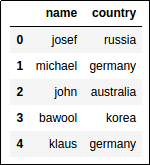BEFORE: original columns 'name' and 'country'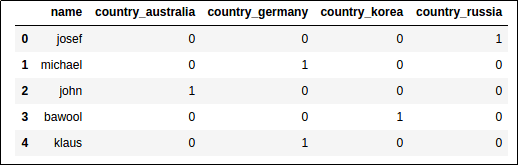AFTER: 'country' column has been replaced
by the derived one-hot columns

## Nulls/NaNs as separate category

Use dummy_na=True:

import pandas as pd
import numpy as np

df = pd.DataFrame({
'country': ['germany',np.nan,'germany','united kingdom','america','united kingdom']
})

pd.get_dummies(df['country'], dummy_na=True)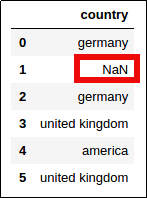In the original dataframe one
of the values is NaN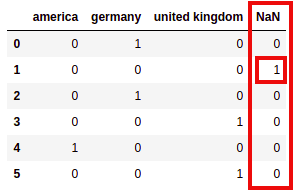If you pass dummy_na=True, you get an extra column for NaNs